# A bar chart 5 ways in ggplot2

Andy, Tom, and ggplot2 walk into a bar…

data visualization
ggplot2
tidyverse
web scraping
Author

Thomas Mock

Published

August 1, 2020

## Credit

Andy Kirk put together Five Ways to present bar charts as part of his `Five ways to...` series back in 2019. The plots below are his original ideas, just recreated in `ggplot2`.

I originally recreated his plots in `ggplot2` and published them as a gist and on Twitter in July 2019, stumbled upon it again recently, and thought why not capture it as a proper blog-post!

Additionally, when I originally made these remakes, `ggplot2` required `coord_flip()` whereas the most recent version of `ggplot2` allows you to natively create horizontal bar charts! I’ve thus changed a little bit of the code from the original gist to reflect the new options in `ggplot2`.

Again thank you to Andy Kirk for the prompt! Make sure to check out his blog in general for all sorts of great data viz tips.

## Source Data

The data comes from Wikipedia, specifically a list of the most streamed songs on Spotify. We can scrape the table into R w/ `rvest`.

``````library(rvest)
library(tidyverse)``````

Now that we have the libraries loaded, let’s read in the data, pull in the top 100, and add some new columns to use across our charts.

``````url <- "https://en.wikipedia.org/wiki/List_of_most-streamed_songs_on_Spotify"

df <- url %>%
html_table(fill = TRUE) %>%
.[] %>%
select(Rank:`Date published`) %>%
set_names(nm = c("rank", "song_name", "streams", "artist", "date_published")) %>%
slice(1:100) %>%
mutate(num_rank = parse_number(rank),
streams_comma = streams,
streams = parse_number(streams)/1000,
streams_text = if_else(
num_rank == 1,
paste(round(streams, digits = 2), "billion streams"),
as.character(round(streams, digits = 2))
),
lab_text = glue::glue("{rank}. {song_name} by {artist}"),
) %>%
as_tibble()

df %>% glimpse()``````
``````Rows: 100
Columns: 9
\$ rank           <chr> "1", "2", "3", "4", "5", "6", "7", "8", "9", "10", "11"…
\$ song_name      <chr> "\"Shape of  You\"", "\"Blinding Lights\"", "\"Dance Mo…
\$ streams        <dbl> 3.109, 2.920, 2.542, 2.403, 2.327, 2.289, 2.268, 2.263,…
\$ artist         <chr> "Ed Sheeran", "The Weeknd", "Tones and I", "Post Malone…
\$ date_published <chr> "28 March 2017", "29 November 2019", "10 May 2019", "15…
\$ num_rank       <dbl> 1, 2, 3, 4, 5, 6, 7, 8, 9, 10, 11, 12, 13, 14, 15, 16, …
\$ streams_comma  <chr> "3,109", "2,920", "2,542", "2,403", "2,327", "2,289", "…
\$ streams_text   <chr> "3.11 billion streams", "2.92", "2.54", "2.4", "2.33", …
\$ lab_text       <glue> "1. \"Shape of  You\" by Ed Sheeran", "2. \"Blinding L…``````

Note that there is `\` in front of the song name and in the lab_text as there we have to escape the `"` in each of those strings.

# Chart 1: Font-height bars

``````font_height_bars <- df %>%
filter(num_rank <=10) %>%
ggplot(aes(y = fct_reorder(lab_text, streams), x = streams)) +
geom_col(fill = "#7dc8c4", width = 0.3) +
theme(text = element_text(family = "Nunito Bold", face = "bold", size = 14),
axis.text = element_text(face = "bold"),
axis.ticks = element_blank(),
panel.grid.minor = element_blank(),
panel.grid.major.y = element_blank(),
panel.grid.major.x = element_line(color = "lightgrey")) +
labs(x = "\nNumber of streams in billions",
y = "") +
scale_x_continuous(limits = c(0,2.700), expand = c(0, 0),
breaks = scales::breaks_pretty(n = 10)
)

ggsave("font_height_bars.png", font_height_bars, dpi = 300,
height = 6, width = 14, units = "in")``````
``Warning: Removed 2 rows containing missing values (position_stack).``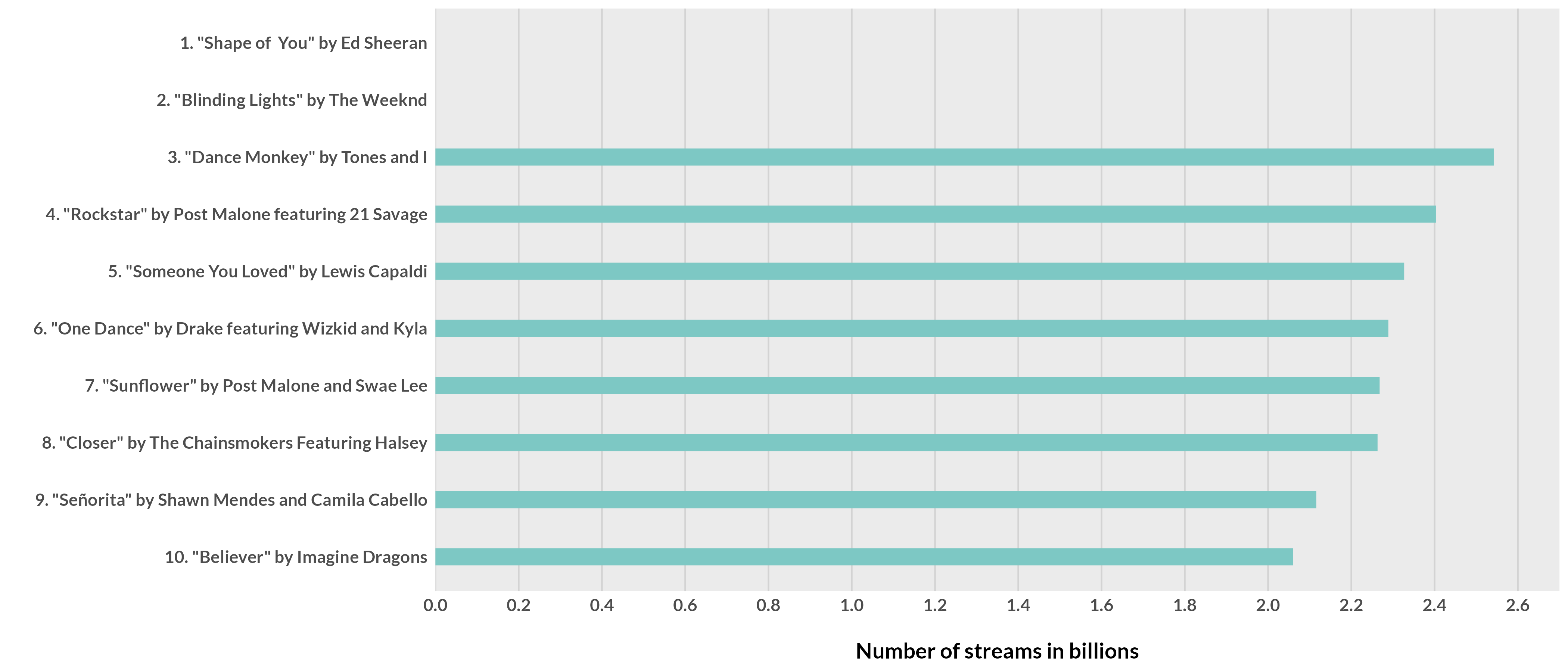# Chart 2: Bars with invisible gridlines

``````invis_gridline <- df %>%
filter(num_rank <=10) %>%
ggplot(aes(x = streams, y = fct_reorder(lab_text, streams))) +
geom_col(fill = "#3686d3", width = .9) +
geom_vline(data = data.frame(x = seq(0, 2.6, .2)),
aes(xintercept = x), color = "white", size = 0.5) +
theme_minimal() +
theme(text = element_text(family = "Nunito Bold", face = "bold", size = 14),
axis.text = element_text(face = "bold"),
axis.ticks = element_blank(),
panel.grid = element_blank()) +
labs(x = "\nNumber of streams in billions",
y = "") +
scale_x_continuous(limits = c(0,2.7), expand = c(0, 0),
breaks = scales::breaks_pretty(n = 10))

ggsave("invis_gridline.png", invis_gridline, dpi = 300,
height = 6, width = 14, units = "in")``````
``Warning: Removed 2 rows containing missing values (position_stack).``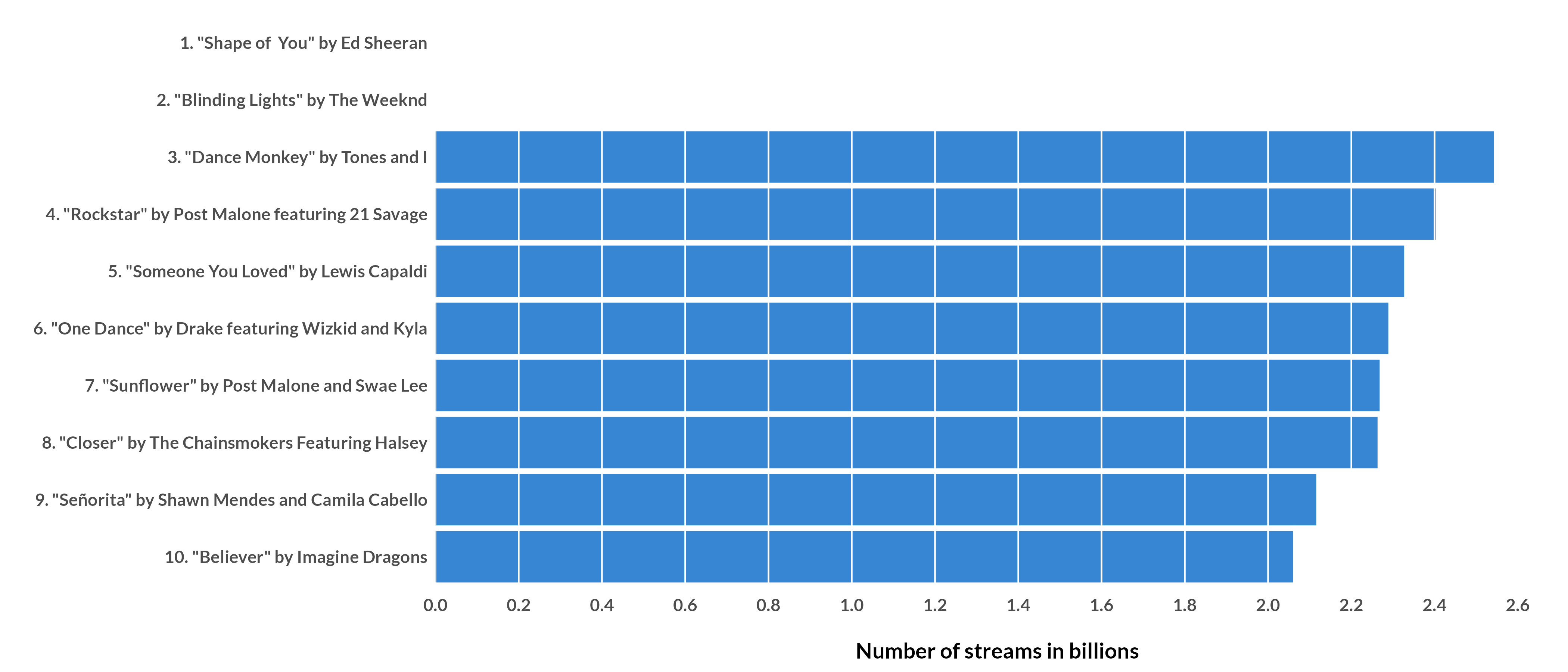# Chart 3: Direct labels

``````direct_label <- df %>%
filter(num_rank <=10) %>%
ggplot(aes(x = streams, y = fct_reorder(lab_text, streams))) +
geom_col(fill = "#303844", width = .9) +
geom_text(aes(y = fct_reorder(lab_text, streams), x = streams, label = streams_text),
color = "white", hjust = 1, fontface = "bold", position = position_nudge(x = -.020)) +
theme_minimal() +
theme(text = element_text(family = "Nunito Bold", face = "bold", size = 16),
axis.text = element_text(face = "bold"),
axis.text.x = element_blank(),
axis.ticks = element_blank(),
panel.grid = element_blank()) +
labs(y = "",
x = "") +
scale_x_continuous(limits = c(0,2.7), expand = c(0, 0),
breaks = scales::breaks_pretty(n = 10))

ggsave("direct_label.png", direct_label, dpi = 300,
height = 6, width = 14, units = "in")``````
``Warning: Removed 2 rows containing missing values (position_stack).``
``Warning: Removed 2 rows containing missing values (geom_text).``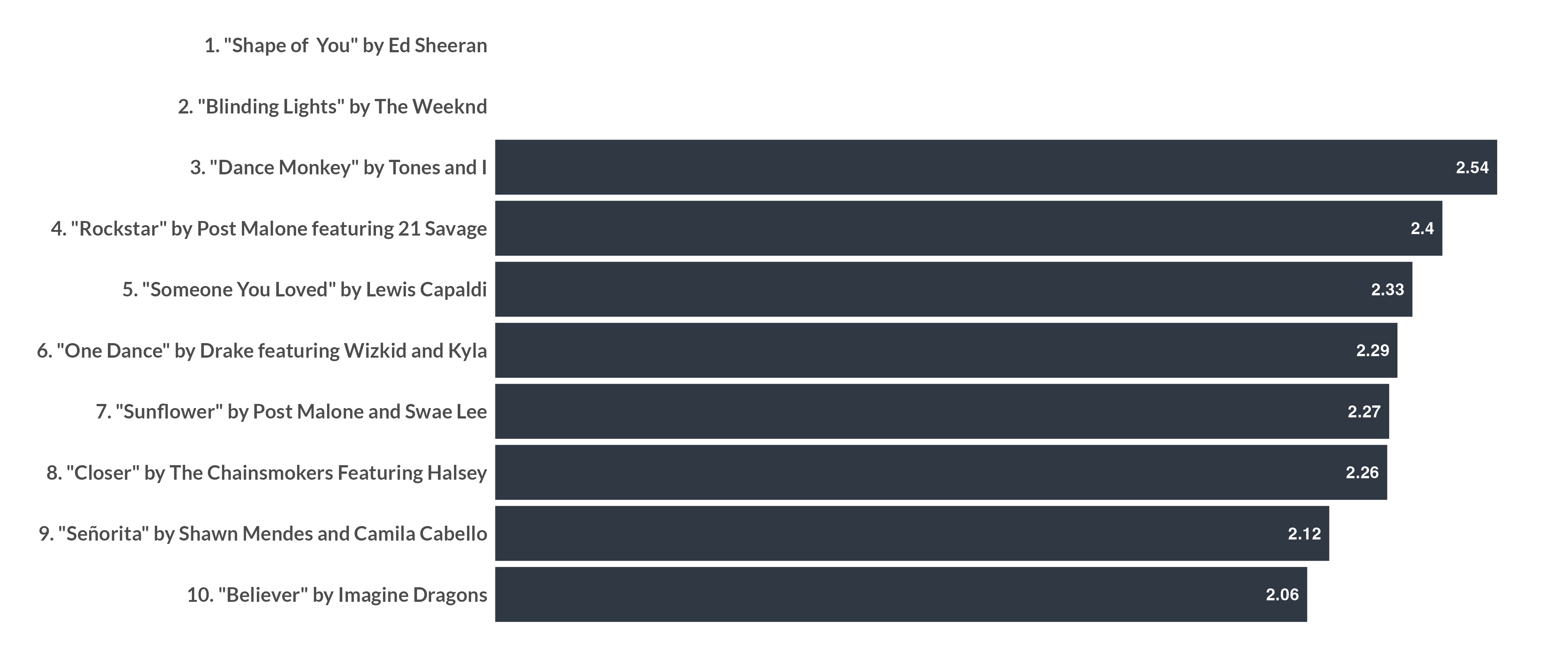# Chart 4: Labels above

``````label_above <- df %>%
filter(num_rank <=10) %>%
ggplot(aes(x = streams, y = fct_reorder(lab_text, streams))) +
geom_col(fill = "#c2545b", width = .2) +
geom_text(aes(x = 0, y = fct_reorder(lab_text, streams),  label = lab_text),
color = "black", hjust = 0, position = position_nudge(y = 0.3),
fontface = "bold", family = "Nunito Bold", size = 4) +
geom_text(aes(x = streams, y = fct_reorder(lab_text, streams), label = streams_text),
color = "#cf7a7f", hjust = 1, position = position_nudge(x = -.02, y = 0.3),
fontface = "bold", family = "Nunito Bold", size = 4) +
theme_minimal() +
theme(text = element_text(family = "Nunito Bold", face = "bold", size = 14),
axis.text = element_blank(),
axis.text.x = element_blank(),
axis.ticks = element_blank(),
panel.grid = element_blank()) +
labs(y = "",
x = "") +
scale_x_continuous(limits = c(0,2.7), expand = c(0, 0),
breaks = scales::breaks_pretty(n = 10))

ggsave("label_above.png", label_above, dpi = 300,
height = 6, width = 14, units = "in")``````
``Warning: Removed 2 rows containing missing values (position_stack).``
``Warning: Removed 2 rows containing missing values (geom_text).``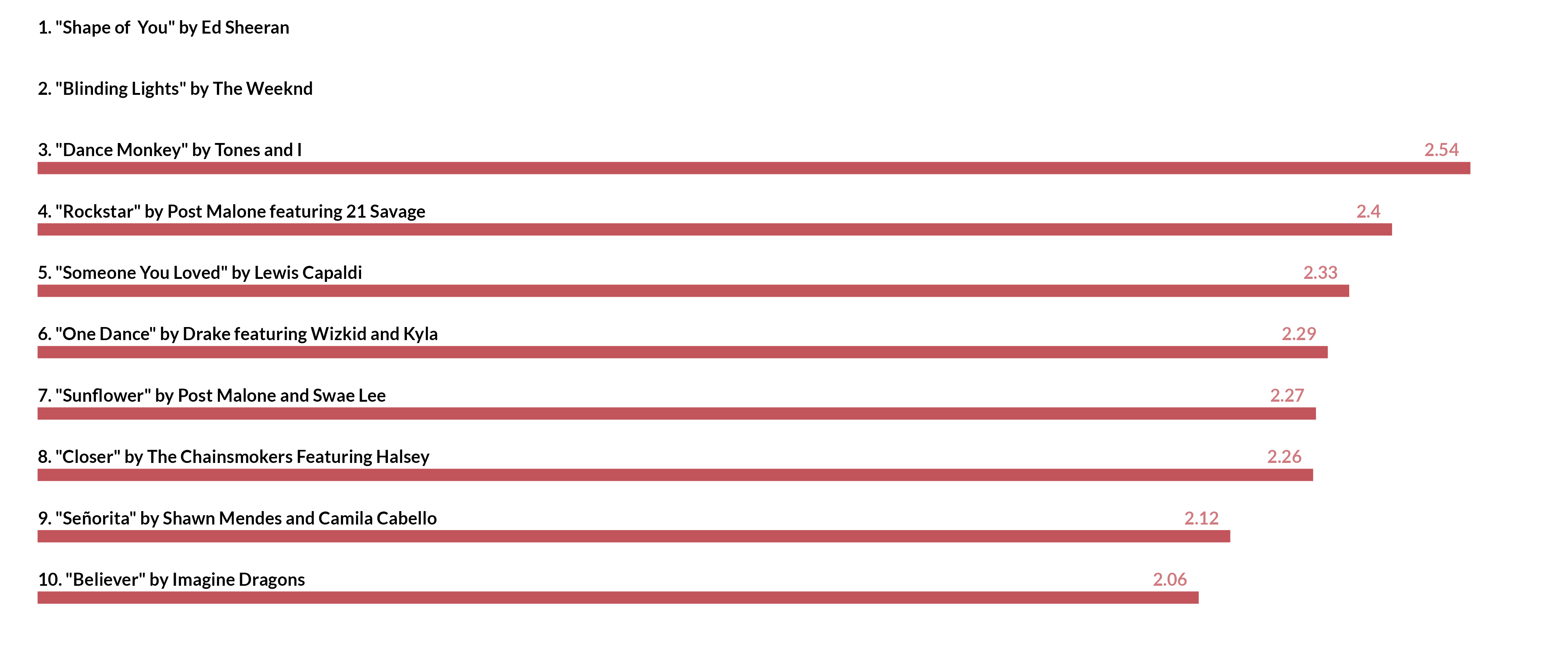# Chart 5: Lollipop

``````lollipop_bar <- df %>%
filter(num_rank <=10) %>%
ggplot(aes(x = streams, y = fct_reorder(lab_text, streams))) +
geom_col(fill = "grey", width = .8) +
geom_point(shape = 21, fill = "orange", color = "black", size = 20, stroke = 1) +
geom_text(aes(x = streams, y = fct_reorder(lab_text, streams), label = streams),
color = "black", hjust = 0.5,
fontface = "bold") +
theme_minimal() +
theme(text = element_text(family = "Nunito Bold", face = "bold", size = 14),
axis.text = element_text(face = "bold"),
axis.text.x = element_blank(),
axis.ticks = element_blank(),
panel.grid = element_blank(),
axis.title.x = element_text(hjust = 0)) +
labs(y = "",
x = "Number of streams in billions") +
scale_x_continuous(limits = c(0,2.7), expand = c(0, 0),
breaks = scales::breaks_pretty(n = 10)) +
NULL

ggsave("lollipop_bar.png", lollipop_bar, dpi = 300,
height = 8, width = 16, units = "in")``````
``Warning: Removed 2 rows containing missing values (position_stack).``
``Warning: Removed 2 rows containing missing values (geom_point).``
``Warning: Removed 2 rows containing missing values (geom_text).``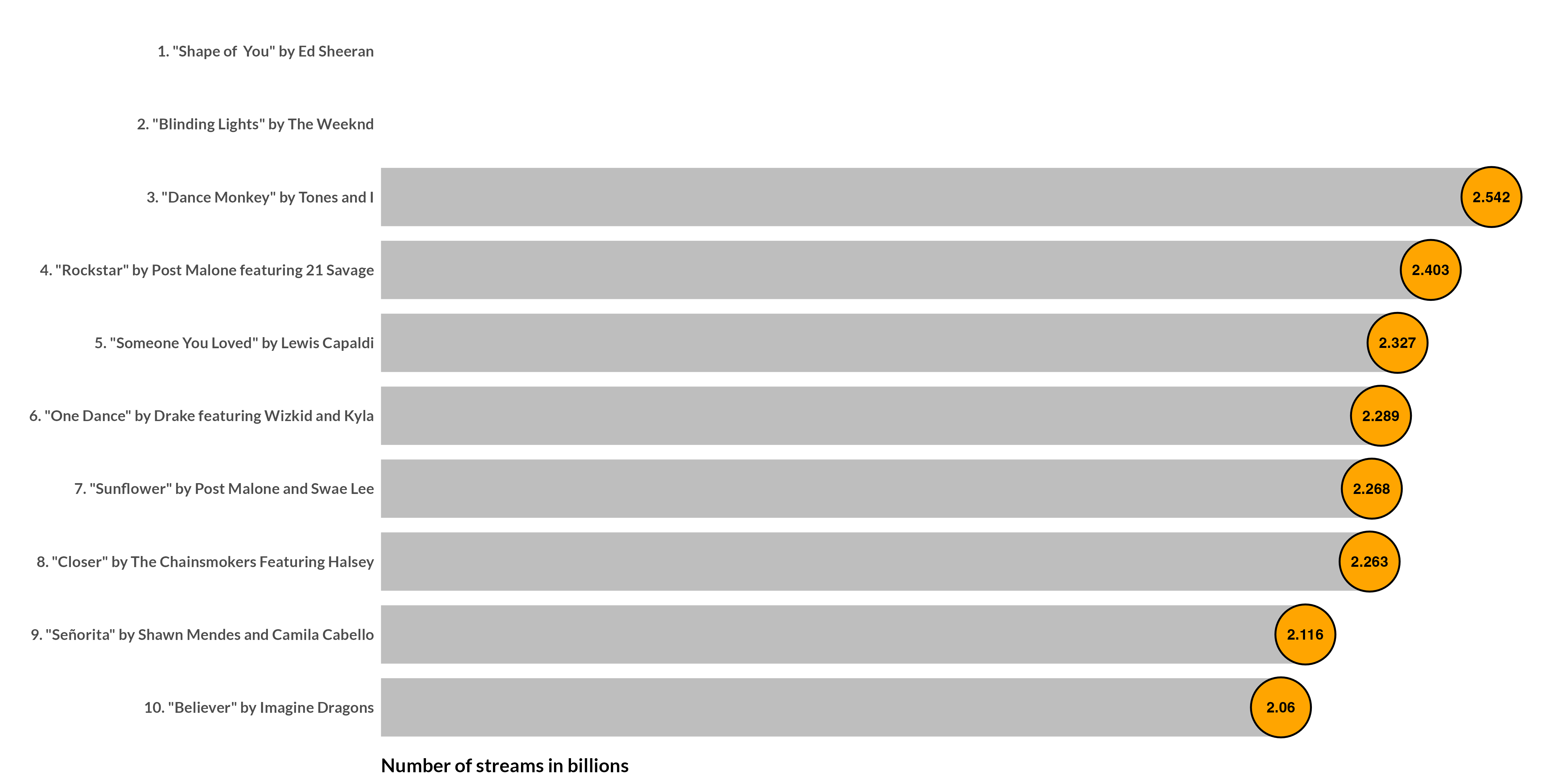``````─ Session info ───────────────────────────────────────────────────────────────
setting  value
version  R version 4.2.0 (2022-04-22)
os       macOS Monterey 12.2.1
system   aarch64, darwin20
ui       X11
language (EN)
collate  en_US.UTF-8
ctype    en_US.UTF-8
tz       America/Chicago
date     2022-04-28
pandoc   2.18 @ /Applications/RStudio.app/Contents/MacOS/quarto/bin/tools/ (via rmarkdown)
quarto   0.9.294 @ /usr/local/bin/quarto

─ Packages ───────────────────────────────────────────────────────────────────
package     * version date (UTC) lib source
dplyr       * 1.0.8   2022-02-08  CRAN (R 4.2.0)
forcats     * 0.5.1   2021-01-27  CRAN (R 4.2.0)
ggplot2     * 3.3.5   2021-06-25  CRAN (R 4.2.0)
purrr       * 0.3.4   2020-04-17  CRAN (R 4.2.0)
readr       * 2.1.2   2022-01-30  CRAN (R 4.2.0)
rvest       * 1.0.2   2021-10-16  CRAN (R 4.2.0)
sessioninfo * 1.2.2   2021-12-06  CRAN (R 4.2.0)
stringr     * 1.4.0   2019-02-10  CRAN (R 4.2.0)
tibble      * 3.1.6   2021-11-07  CRAN (R 4.2.0)
tidyr       * 1.2.0   2022-02-01  CRAN (R 4.2.0)
tidyverse   * 1.3.1   2021-04-15  CRAN (R 4.2.0)

 /Library/Frameworks/R.framework/Versions/4.2-arm64/Resources/library

──────────────────────────────────────────────────────────────────────────────``````